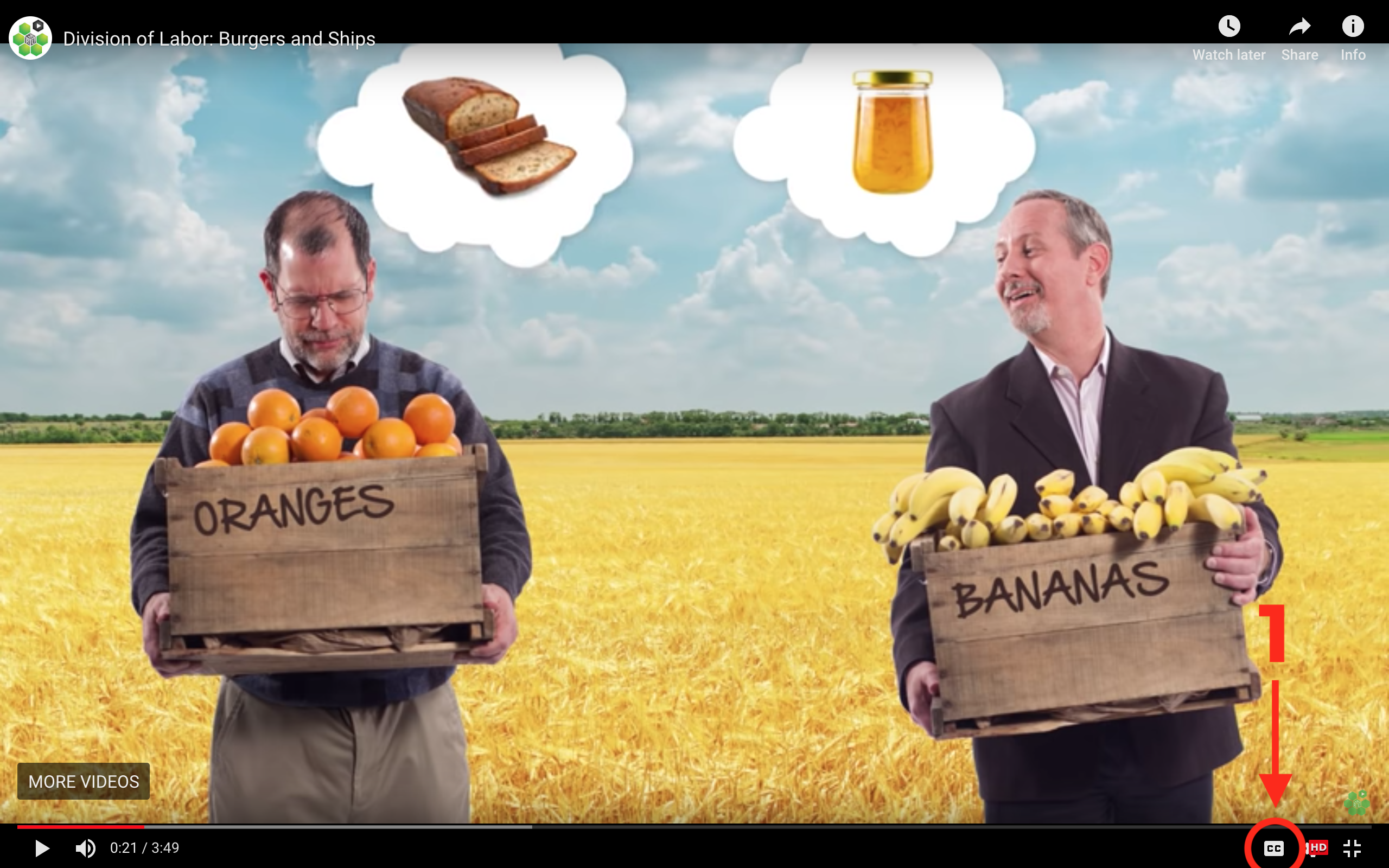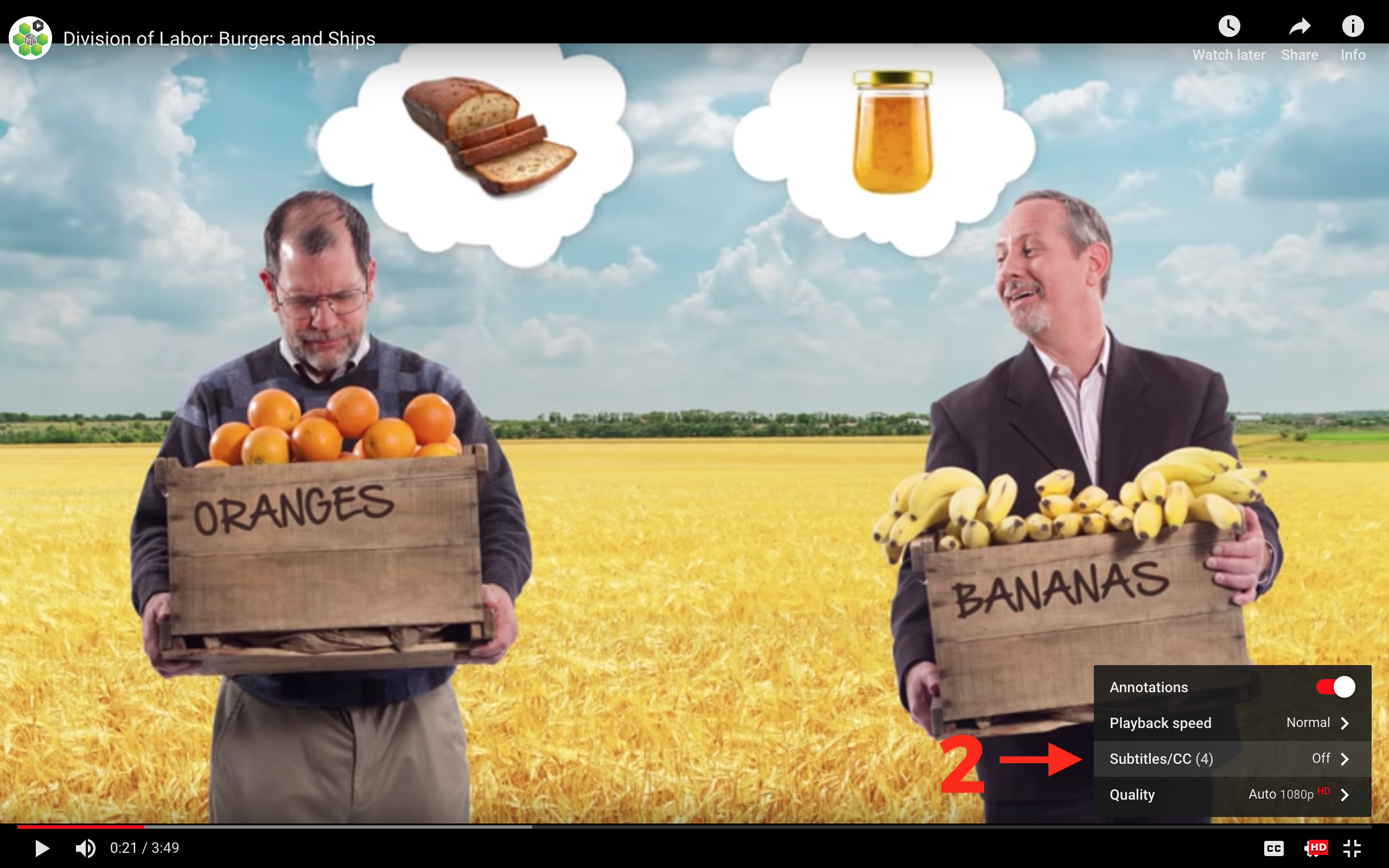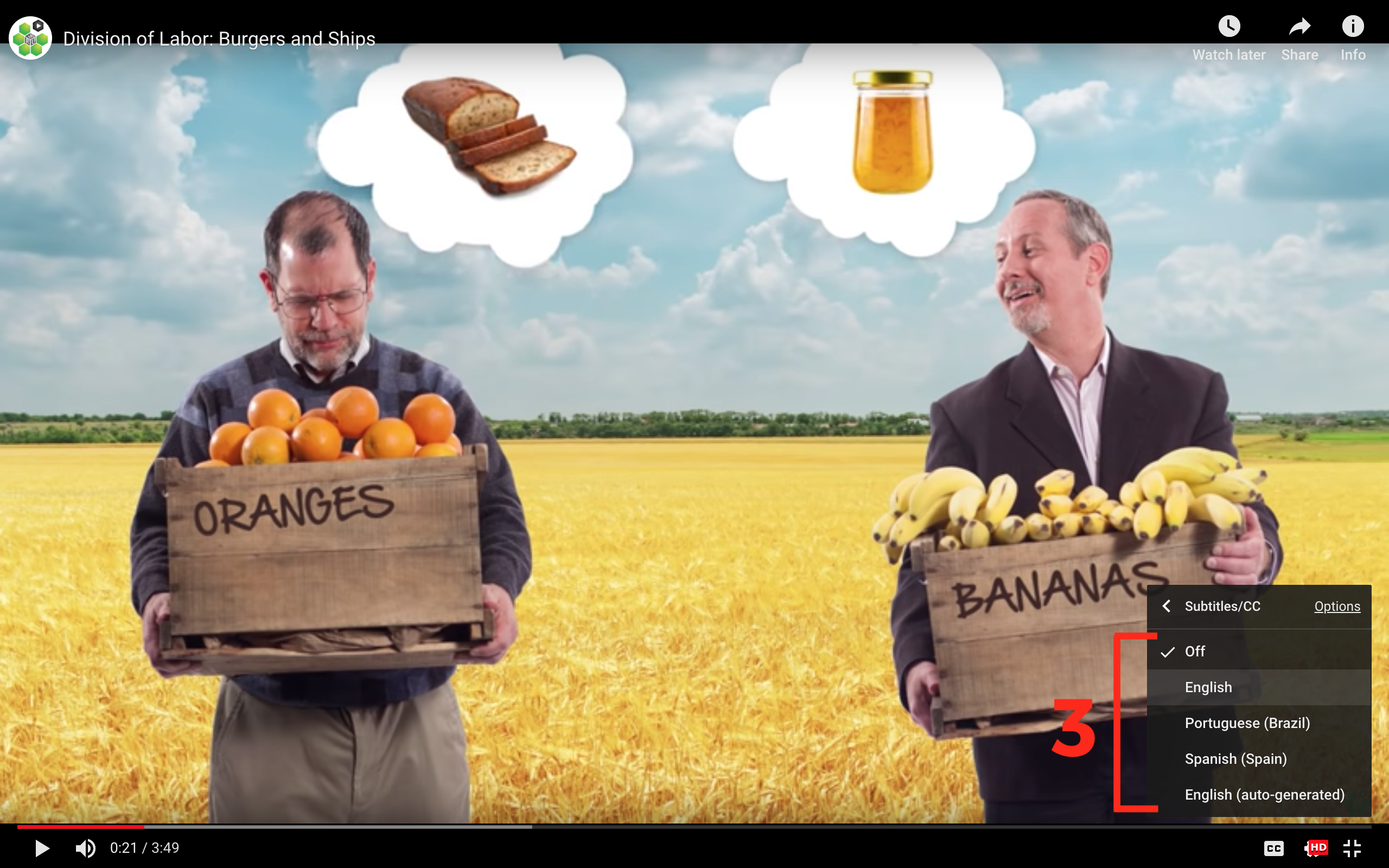## Office Hours: The Solow Model

In last week’s Principles of Macroeconomics video, you learned about the steady state level of capital and the Solow model of economic growth. Here are two of the practice questions from that video:

Country A has K=10,000 and produces GDP according to the following equation: GDP=5√K. 1) If the country devotes 25% of its GDP to making investment goods, how much is the country investing? 2) If 1% of all machines become worthless every year (they depreciate, in other words) in Country A, GDP is...?

These are tricky problems! If you're stumped, don’t worry. Mary Clare Peate from the Marginal Revolution University team is here to help.

## Transcript

Today, we're gonna solve the following problem from our video on the Solow Model's steady state. Country A produces GDP according to the following equation: GDP equals five times the square root of K and has a capital stock of 10,000. If the country devotes 25% of its GDP to making investment goods, how much is this country investing? Additionally, if 1% of all capital depreciates every year, is the country's GDP increasing, decreasing, or remaining constant in that steady state? As always, it's best to watch the video first and try to solve this problem by yourself. If you have remaining questions, you can always return, and we'll work through the problem together.

Ready? This question has two parts. First, finding how much this country is investing, and second, determining whether or not its GDP is growing. Fortunately, that first question is actually a necessary step for solving the second one. First things first. The relevant information from the problem is in that top right-hand corner of the board for reference. As always, it's best to identify steps for solving the problem. The first of the two questions is fairly straightforward. Simply derive the investment equation from the GDP equation and then solve for I, given the current capital stock of 10,000.

To solve the second question, we'll need our answer from question one: the amount of capital we're accumulating through investment. We'll then find out how much capital we're losing to depreciation, and finally, we'll compare the two, investment to depreciation to determine whether the country's capital stock, and therefore, its GDP, is increasing, decreasing, or remaining constant in the steady state.

Let's look a bit more in depth at this problem by graphing it. As you can see, GDP is measured on the y-axis. In previous Solow questions, you may have seen this labeled as total output or Y instead of GDP. And K, physical capital, is measured on the x-axis We know that this country's GDP is five times the square root of K, and we've actually already graphed it. This equation shows that GDP is a function of K. As K increases, GDP also increases, albeit by a smaller amount because of the law of diminishing returns.

It's also worth noting that we're actually holding other variables that could affect GDP constant. Things like education, or population, and ideas. So increasing capital is the only way this country's GDP grows. In our example, this country has \$10,000 dollars’ worth of capital. If we plug that into equation, GDP is 500. Now we know that GDP is five times the square root of K. And we also know that Investment is 25% of GDP, therefore, we can substitute five times the square root of K in for GDP. And that's it, for step one.

To take a short cut, since we know GDP in this instance is 500, 25% of 500 is 125. This country is investing \$125 dollars into capital accumulation. And that's the answer to step two. A few quick things to note here. Several variables are actually measured along the y-axis. Not just GDP, but we're also measuring investment, and eventually we're going to add depreciation. In general, it looks pretty cluttered if we were to add all of those labels up to the top. So, we'll just leave it at GDP.

And one other thing to note: if we're investing 125, and total GDP is 500, what's happened to that remaining GDP? It's being used for consumption, you know, buying stuff. One of the follow-up questions at the end of this video actually tests your understanding of this. So, while this country is accumulating 125 worth of capital, we don't yet know if the country's capital stock overall is increasing, decreasing, or remaining constant, because we don't know how much of the capital stock is wearing down, or depreciating.

In the real world, machines break, laptops die. Think of physical capital in your own life. How many times have you dropped your iPhone and had to get a new one? Or how often have you replaced an old phone, even though it still worked. So even though capital is being added to the stock of 10,000 through investment, some of this 10,000 is also being lost to depreciation, to those iPhones dropping. It helps to graph depreciation. We know from the initial problem that 1% of all capital stock is depreciating. Graphically, 1% times K can be represented roughly like this: If capital stock is 10,000, 1% of 10,000 is 100. So, 100 dollars’ worth of capital stock is wearing down, or depreciating, each year.

We've now solved for step 3. We now have investment and depreciation, and can compare the two. If the country invests 125 worth of capital, and loses 100 to depreciation, then investment is greater than depreciation, and therefore, the capital stock will grow by 25 this year, as represented by the difference between these two curves. We can now answer that final question. The country's capital stock is increasing, and therefore, so too is GDP. And that's our answer. Because remember, according to the equation increases in K, increase GDP. As long as investment is greater than depreciation K and GDP will continue to increase until the country's capital investment equals depreciation. At this point, it reaches steady state because capital gain through investment is perfectly offset to capital lost from depreciation. And therefore, neither the capital stock nor GDP changes at this point.

## Subtitles

Verified Available Languages
• English
• Spanish
• Chinese
• Hindi
• French
• Arabic

### Turn captions on or off:

1. If captions are available the (CC) icon will be visible on the player.
2. To turn captions on, tap (CC).
3. To turn captions off, tap (CC) again.### Select caption language:

1. Click the settings icon (⚙) at the bottom of the video screen.
2. Click Subtitles/CC.
3. Select a language.## Contribute Translations!

Join the team and help us provide world-class economics education to everyone, everywhere for free! You can also reach out to us at support@mru.org for more info.

## Accessibility

We aim to make our content accessible to users around the world with varying needs and circumstances.

Currently we provide:

Are we missing something? Please let us know at support@mru.org